Request your copy of SimphoSOFT® now

Webinars
Product brochureApplication notesThe slowly varying envelope approximation (SVEA) for calculating and modeling light propagation can be used to simplify the scalar form of Maxwell’s equations that describe the propagation of electromagnetic waves through a material or vacuum. SVEA is valid only in a narrow range of propagation angles.

In the scalar form of Maxwell’s equations, the electric field can be written as

 E(x,y,z,t) = φ(x,y,z,t) exp[ -iωt ] + c.c.

However, the phase of φ(x,y,z,t) varies rapidly along the propagation direction (usually specified as the z-direction). This rapid phase variation is not important for many types of problems and can be removed by introducing a slowly varying field A(x,y,z,t) and constant k, such that

 φ(x,y,z,t) = A(x,y,z,t) exp[ ikz ]

resulting in the following SVEA

 E(x,y,z,t) = A(x,y,z,t) exp[ ikz -iωt ] + c.c.

It is assumed that A(x,y,z,t) varies slowly in the propagation direction z as well as the other two directions and time t,

 | ∂A/∂x | << k | A | | ∂A/∂y | << k | A | | ∂A/∂z | << k | A | | ∂A/∂t | << ω | A |

which implies, for example, that

 ∂2A/∂z2 ≈ 0

This reduces a second order differential equation to a first order equation that is easier to solve. The resulting form of the first-order scalar Maxwell’s equations is called the slowly varying envelope approximation (or the paraxial approximation, if the back-propagating term A*(x,y,z,t) is left out, since it is valid only in a narrow range of angles).

 References  P. N. Butcher and D. Cotter, The Elements of Nonlinear Optics, Cambridge U. Press (1990).SimphoSOFT® mathematical model is based on SVEA.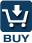SimphoSOFT® can be purchased as a single program and can be also configured with Energy Transfer add-on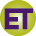, Multi-Beam add-on, Optimization add-on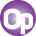, Z-scan add-on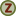, and MPA Info+ add-onfor an additional charge. Please, contact our sales staff for more information e-mail phone: +1 (973) 621-2340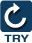You can request a remote evaluation of our software by filling out online form -- no questions asked to get it, no need to install it on your computer.